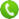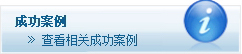# C#性能优化5大技巧

1、你是否用异常机制来处理过用户输入验证？

`class BusinessLogcCheck`
`{`
`    public void Check()`
`    {`
`        try`
`        {`
`            //Your validation code is here`
`           `
`        }`
`        catch (Exception ex)`
`        {`
`            throw new Exception("My own exception");`
`        }`
` `
`    }`
`}`

`using System;`
`using System.Collections.Generic;`
`using System.Linq;`
`using System.Text;`
`using System.Diagnostics;`
`using System.IO;`
`using System.Net;`
`using System.Net.NetworkInformation;`
`namespace Test1`
`{`
`    class Program`
`    {`
`        public static void ThrowTest()`
`        {`
`            throw new Exception("This is exceptopn");`
`        }`
`        public static Boolean Return()`
`        {`
`            return false;`
`        }`
` `
`        static void Main(string[] args)`
`        {`
` `
`            Stopwatch sw = new Stopwatch();`
`            sw.Start();`
` `
`            try`
`            {`
`                    ThrowTest();`
`            }`
`            catch`
`            {`
`               `
`            }`
`            sw.Stop();`
`            Console.WriteLine("With Exception " + sw.ElapsedTicks);`
` `
`            sw.Restart();`
`            try`
`            {`
`                Return();`
`            }`
`            catch`
`            {`
` `
`            }`
`            sw.Stop();`
`            Console.WriteLine("With Return " + sw.ElapsedTicks);`
`            Console.ReadLine();`
`        }`
`    }`
`}`2.绝对不要在循环中使用try-Catch.

`using System;`
`using System.Collections.Generic;`
`using System.Linq;`
`using System.Text;`
`using System.Diagnostics;`
`using System.IO;`
`using System.Net;`
`using System.Net.NetworkInformation;`
`namespace Test1`
`{`
`    class Program`
`    {`
`        static void Method1()`
`        {`
`            for (int i = 0; i < 1000; i++)`
`            {`
`                try`
`                {`
`                    int value = i * 100;`
`                    if (value == -1)`
`                    {`
`                        throw new Exception();`
`                    }`
`                }`
`                catch`
`                {`
`                }`
`            }`
`        }`
`        static void Method2()`
`        {`
`            try`
`            {`
`                for (int i = 0; i < 1000; i++)`
`                {`
`                    int value = i * 100;`
`                    if (value == -1)`
`                    {`
`                        throw new Exception();`
`                    }`
`                }`
`            }`
`            catch`
`            {`
`            }`
`        }`
` `
`        static void Main(string[] args)`
`        {`
` `
`            Stopwatch sw = new Stopwatch();`
`            sw.Start();`
`            Method1();`
`            sw.Stop();`
` `
`            Console.WriteLine("Within Loop " + sw.ElapsedTicks);`
` `
`            sw.Restart();`
`            Method2();`
`            sw.Stop();`
`            Console.WriteLine("Outside of Loop " + sw.ElapsedTicks);`
`            Console.ReadLine();`
`        }`
`    }`
`}`3.你是否疯狂了，以致于使用new操作符来创建整型变量?

`using System;`
`using System.Collections.Generic;`
`using System.Linq;`
`using System.Text;`
`using System.Diagnostics;`
`using System.IO;`
`using System.Net;`
`using System.Net.NetworkInformation;`
`namespace Test1`
`{`
`    class Program`
`    {`
`        static void Main(string[] args)`
`        {`
`            Stopwatch sw = new Stopwatch();`
`            sw.Start();`
`            for (int i = 0; i < 1000; i++)`
`            {`
`                int a = new int();`
`                a = 100;`
`            }`
`            sw.Stop();`
`            Console.WriteLine("Using New operator:- " + sw.ElapsedTicks);`
`            sw.Restart();`
`            for (int i = 0; i < 1000; i++)`
`            {`
`                int a;`
`                a = 100;`
`            }`
`            sw.Stop();`
`            Console.WriteLine("Without new operator:- "+ sw.ElapsedTicks);`
`            Console.ReadLine();`
`        }`
`    }`
`}`OK！如果你的程序是web程序呢？如果你要校验流行的web程序的点击数，我确定每天将会超过1000个。

4.根据目的选择最好的集合。

`using System;`
`using System.Collections.Generic;`
`using System.Linq;`
`using System.Text;`
`using System.Diagnostics;`
`using System.IO;`
`using System.Net;`
`using System.Net.NetworkInformation;`
`namespace Test1`
`{`
`    class Program`
`    {`
`        static void Main(string[] args)`
`        {`
`            List<Int32> li = new List<Int32>(1000);`
`            Dictionary<int, int> di = new Dictionary<int, int>(1000);`
`            int[] arr = new int;`
`            int a;`
`           `
`            for (int i = 0; i < 1000; i++)`
`            {`
`                li.Add(i);`
`                di.Add(i, i);`
`                arr[i] = i;`
`            }`
` `
`            Stopwatch sw = new Stopwatch();`
`            sw.Start();`
`            a = li;`
`            sw.Stop();`
`            Console.WriteLine("From list:- " + sw.ElapsedTicks);`
` `
` `
`            sw.Start();`
`            a = arr;`
`            sw.Stop();`
`            Console.WriteLine("From Integer array:- " + sw.ElapsedTicks);`
` `
` `
`            sw.Restart();`
`            a = di;`
`            sw.Stop();`
`            Console.WriteLine("From Dictionary:- " + sw.ElapsedTicks);`
`            Console.ReadLine();`
`        }`
`    }`
`}`5.函数很好，但有时却不时。

`using System;`
`using System.Collections.Generic;`
`using System.Linq;`
`using System.Text;`
`using System.Diagnostics;`
`using System.IO;`
`using System.Net;`
`using System.Net.NetworkInformation;`
`namespace Test1`
`{`
`    class test`
`    {`
`        public static void Print()`
`        {`
`            Console.WriteLine("I am function from Class");`
`        }`
`    }`
` `
` `
`    class Program`
`    {`
`        static void Main(string[] args)`
`        {`
`            Stopwatch sw = new Stopwatch();`
`            sw.Start();`
`            test.Print();`
`            sw.Stop();`
`            Console.WriteLine(sw.ElapsedTicks);`
` `
`            sw.Restart();`
`            Console.WriteLine("I am single statement within main");`
`            sw.Stop();`
`            Console.WriteLine(sw.ElapsedTicks);`
` `
`            Console.ReadLine();`
`        }`
`    }`
`}`020-82038459020-82038459